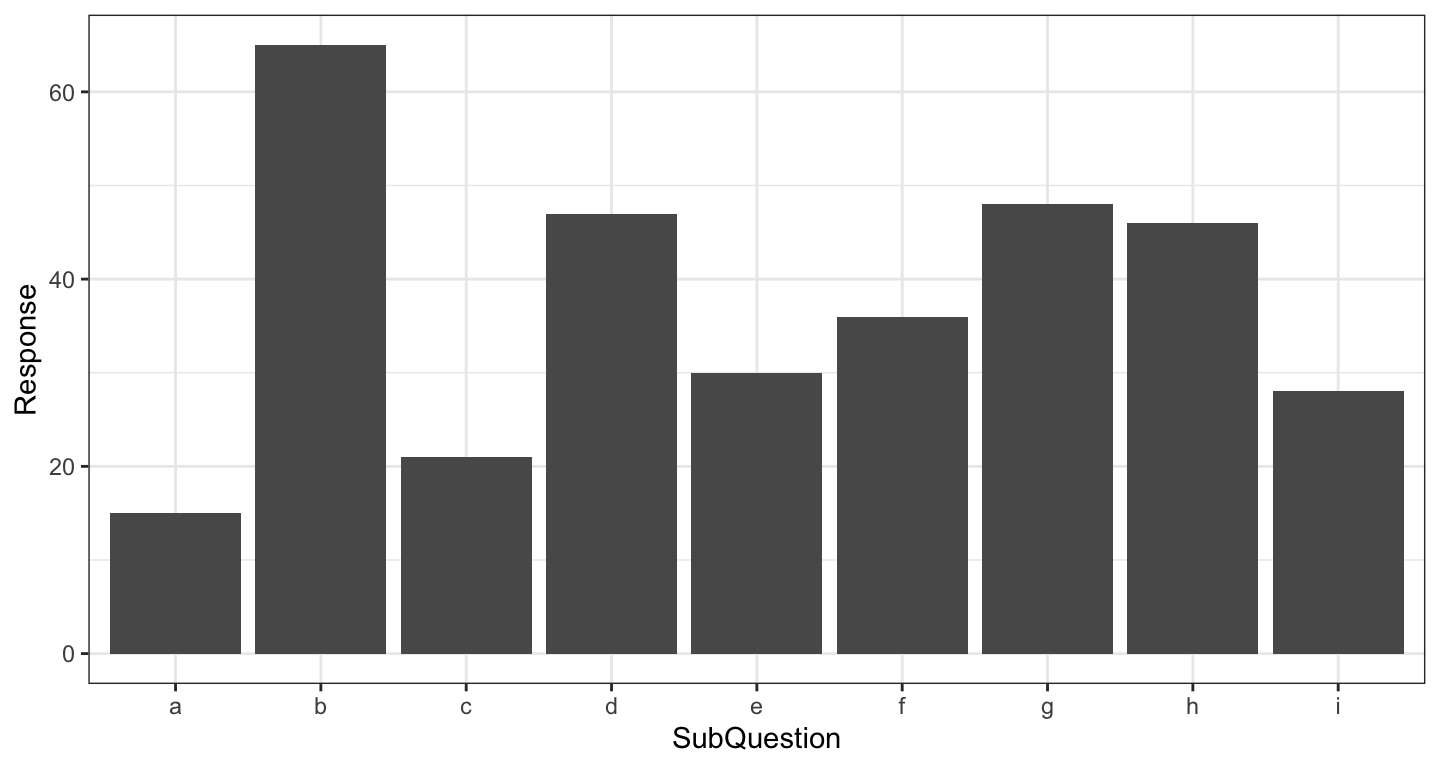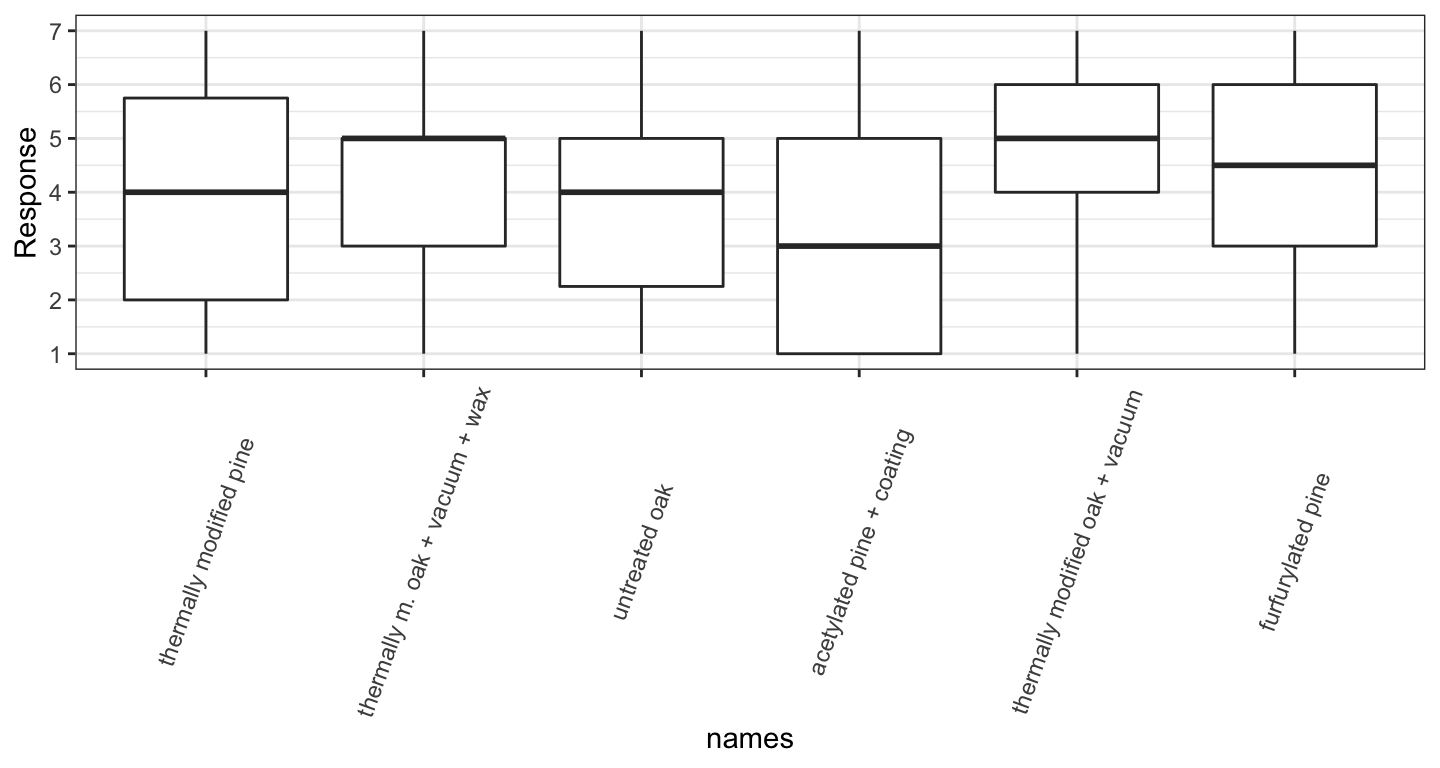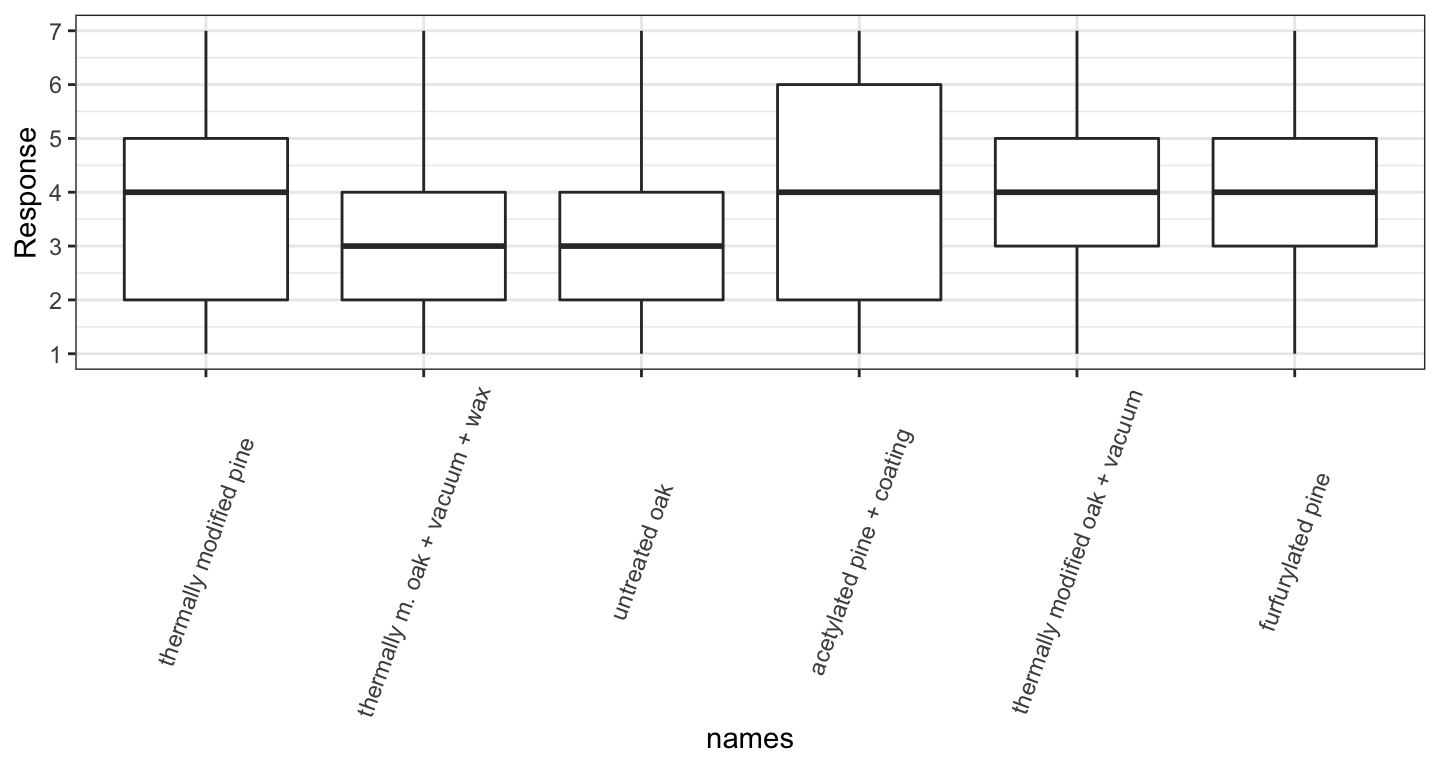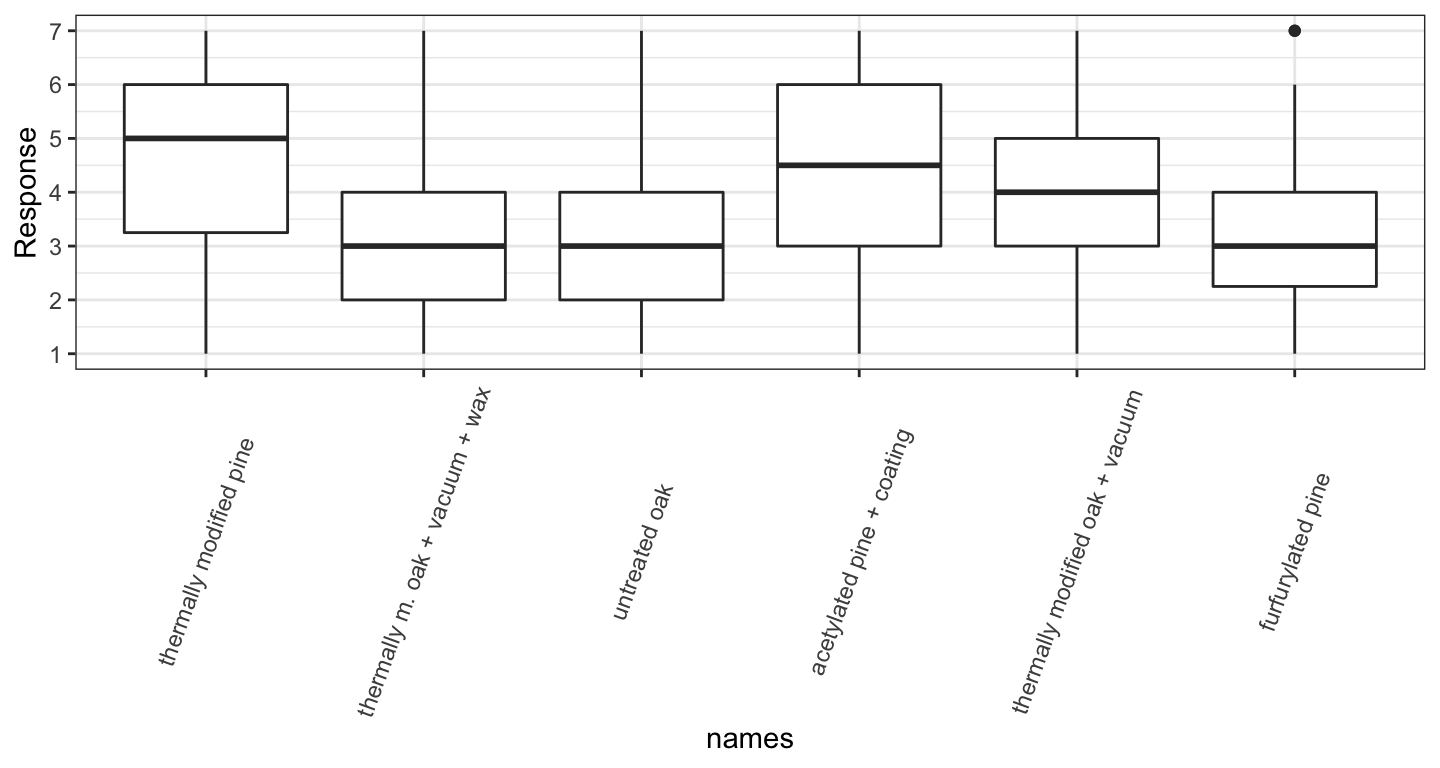# Introduction

This file contains the processing steps associated with a master’s student thesis submitted to UP FAMNIT.

The purpose of the thesis was to assess consumer preferences for wood facade materials in their fresh state and after they have weathered for some time.

Interviews were conducted to gather consumer preferences and material properties were measured in the lab.

The necessary data inputs are uploaded along with this file, with the exception of the individual files containing colour measurements that were combined into a single file for further analysis. The combined file is uploaded, instead.

See the masters thesis for more information about the project, the methods, and the data description. Hypotheses are paraphrased in this document.

# Data

Import and clean survey data. Add supplemental information about samples (material properties).

``````d <- read_csv("data/remesova_ms_data_v1.csv")
colnames(d)[3:13] <- paste("q1_", colnames(d)[3:13], sep="")
colnames(d)[14:19] <- paste("q2_", colnames(d)[14:19], sep="")
colnames(d)[20:25] <- paste("q3_", colnames(d)[20:25], sep="")
colnames(d)[26:31] <- paste("q4_", colnames(d)[26:31], sep="")
colnames(d)[32:37] <- paste("q5_", str_sub(colnames(d)[32:37], 0,2), sep="")
colnames(d)[38:43] <- paste("q6_", str_sub(colnames(d)[38:43], 0,2), sep="")
colnames(d)[44:48] <- paste("q7_", str_sub(colnames(d)[44:48], 0,2), sep="")
colnames(d)[53:56] <- paste("q10_", str_sub(colnames(d)[53:56], 0,2), sep="")
colnames(d) <- "Gender"

d\$Gender <- if_else(d\$Gender == "babyYoda", "Not Specified", d\$Gender)

d.long <- d %>%
pivot_longer(c(50:52), names_to="Region", values_to="Response") %>%
filter(!is.na(Response)) %>%
select(-Response)

d.long <- d.long %>%
pivot_longer(c(44:48), names_to="Age", values_to="AgeGroup") %>%
filter(!is.na(AgeGroup)) %>%
select(-AgeGroup)

d.long <- d.long %>% select(-c(12:13,45:48))

d.long <- d.long %>% pivot_longer(
c(3:41), names_to="Question", values_to="Response") %>%
separate(Question, into=c("Question", "SubQuestion"), remove=FALSE)

age <- tibble(col_name = c("q7_a_", "q7_b_", "q7_c_", "q7_d_", "q7_e_"),
age_group = c("18-25", "26-37", "38-50", "51-64", "65+"))

key <- tibble(code = c("A", "B", "C", "D", "E", "H",
"I", "L", "F", "K", "G", "J",
"AI", "BL", "CF", "DK", "EG", "HJ"),
names = rep(c("thermally modified pine",
"thermally m. oak + vacuum + wax",
"untreated oak",
"acetylated pine + coating",
"thermally modified oak + vacuum",
"furfurylated pine"), times=3))

d.q1 <- d.long %>% filter(Question == "q1")

d.long <- d.long %>%
filter(Question != "q1")

d.long <- left_join(d.long, key, by=c("SubQuestion" = "code"))
d.long <- left_join(d.long, age, by=c("Age" = "col_name")) %>%
mutate(names = fct_relevel(names, "thermally modified pine",
"thermally m. oak + vacuum + wax",
"untreated oak",
"acetylated pine + coating",
"thermally modified oak + vacuum",
"furfurylated pine"))

colour <- left_join(colour, key, by=c("Sample" = "code")) %>%
mutate(state = rep(c("Fresh", "Weathered"), each=6))
colour_change <- left_join(colour_change, key, by = c("Sample" = "code"))``````

## Color data

Import and merge color data.

** NOT RUN Use combined file: remesova_combined_colour_raw_v1.csv **

``````files <- dir_ls("data/color/", glob="*.txt")

quick_parse <- function(file) {
tmp <- read_tsv(file, locale=locale(decimal_mark = ","),
col_names=c("L", "a", "b")) %>%
mutate(blrp = str_sub(file, -22,-15)) %>%
separate(blrp, c("id", "stage"))
}

d.clr <- map_dfr(files, quick_parse)

#write the color data in one file
#write_csv(d.clr, "data/remesova_combined_colour_raw_v1.csv")``````

# Question 1

Overview figure.

``````ggplot(data=d.q1, aes(y=Response, x=SubQuestion)) + theme_bw() +
geom_col()``````
``## Warning: Removed 330 rows containing missing values (position_stack).``# Question 2

Overview figures and summary table.

``````d.q2 <- d.long %>% filter(Question == "q2")

ggplot(data=d.q2, aes(y=Response, x=names)) + theme_bw() +
geom_boxplot() +
scale_y_continuous(breaks=seq(1,7,1)) +
theme(axis.text.x = element_text(angle=70, vjust=0.5))````````````d.q2 %>% group_by(SubQuestion) %>%
summarise(mean = mean(Response, na.rm=TRUE),
median= median(Response, na.rm=TRUE),
mode = Mode(Response),
sd = sd(Response, na.rm=TRUE))``````
``## `summarise()` ungrouping output (override with `.groups` argument)``
``````## # A tibble: 6 x 5
##   SubQuestion  mean median  mode    sd
##   <chr>       <dbl>  <dbl> <dbl> <dbl>
## 1 A            3.93    4       2  1.87
## 2 B            4.30    5       5  1.57
## 3 C            3.73    4       5  1.62
## 4 D            3.14    3       1  1.95
## 5 E            4.51    5       5  1.47
## 6 H            4.22    4.5     6  1.70``````

# Question 3

Overview figures and summary table.

``````d.q3 <- d.long %>% filter(Question == "q3")

ggplot(data=d.q3, aes(y=Response, x=names)) + theme_bw() +
geom_boxplot() +
scale_y_continuous(breaks=seq(1,7,1)) +
theme(axis.text.x = element_text(angle=70, vjust=0.5))````````````d.q3 %>% group_by(names) %>%
summarise(mean = mean(Response, na.rm=TRUE),
median= median(Response, na.rm=TRUE),
mode = Mode(Response),
sd = sd(Response, na.rm=TRUE))``````
``## `summarise()` ungrouping output (override with `.groups` argument)``
``````## # A tibble: 6 x 5
##   names                            mean median  mode    sd
##   <fct>                           <dbl>  <dbl> <dbl> <dbl>
## 1 thermally modified pine          3.99      4     6  1.72
## 2 thermally m. oak + vacuum + wax  3.43      3     4  1.54
## 3 untreated oak                    3.22      3     2  1.45
## 4 acetylated pine + coating        3.88      4     1  2.08
## 5 thermally modified oak + vacuum  3.82      4     3  1.47
## 6 furfurylated pine                3.96      4     5  1.68``````

# Question 4

Overview figures and summary table, basi

``````d.q4 <- d.long %>% filter(Question == "q4") %>%
mutate(Resp.ord = factor(Response, levels=c(1,2,3,4,5,6,7), ordered=TRUE))

ggplot(data=d.q4, aes(y=Response, x=names)) + theme_bw() +
geom_boxplot() +
scale_y_continuous(breaks=seq(1,7,1)) +
theme(axis.text.x = element_text(angle=70, vjust=0.5))````````````d.q4.sum <- d.q4 %>% group_by(names) %>%
summarise(mean = mean(Response, na.rm=TRUE),
median= median(Response, na.rm=TRUE),
sd = sd(Response, na.rm=TRUE),
mode=Mode(Response))``````
``## `summarise()` ungrouping output (override with `.groups` argument)``
``d.q4.sum``
``````## # A tibble: 6 x 5
##   names                            mean median    sd  mode
##   <fct>                           <dbl>  <dbl> <dbl> <dbl>
## 1 thermally modified pine          4.61    5    1.59     5
## 2 thermally m. oak + vacuum + wax  3.03    3    1.39     3
## 3 untreated oak                    3.15    3    1.52     2
## 4 acetylated pine + coating        4.28    4.5  2.04     7
## 5 thermally modified oak + vacuum  3.70    4    1.49     3
## 6 furfurylated pine                3.5     3    1.58     3``````

# Question 5

``````d.q5 <- d.long %>% filter(Question == "q5")

ggplot(data=d.q5, aes(y=Response, x=names)) + theme_bw() +
geom_boxplot() +
scale_y_continuous(breaks=seq(1,7,1)) +
theme(axis.text.x = element_text(angle=70, vjust=0.5))``````